# Power Meter Measurements

Click here to go to a page on linear interpolation for Excel

We have just recently launched a forum for metrologists who calibrate RF power sensors. The goal is to provide detailed help with various topics that our customers encounter throughout the entire calibration process. That leads us to end up supporting not only our products but also equipment from all of the major manufacturers. We would like to encourage the forum to grow.

TEGAM supplied us with their application note on calculating a calibration factor, which appears lower down on this page. Click here to jump down!

The power meter is one of the most useful pieces of test equipment in the microwave laboratory. Learn it well and you will be able to characterize all manner of nonlinear circuit power transfer characteristics, such as amplifiers, mixers, limiters, etc. You can also measure insertion loss (or gain) and return loss of microwave networks, similar to using a scalar analyzer, but at a fraction of the equipment cost. You can find used HP power meters and power sensors on E-bay for just a few hundred bucks, thanks to the continuously tanking wireless industry.

In this chapter of Microwaves101 you will learn the most convenient and accurate way to measure defined compression points (P1dB, P2dB, etc.) of devices such as amplifiers and mixers, using a surprisingly simple technique. You will learn how to measure and plot data such as:

1. Output power gain and efficiency of an amplifier versus input power at fixed frequency
2. One dB compression of an amplifier versus frequency, at different bias points
3. One dB compression of a mixer versus frequency, at different LO powers.

By the way, we are discussing CW or average power meter measurements here. We will describe the use of peak power meters at a later time. If you are interested in peak power measurements or any other topic, please drop us a line!

Power meter basics

Calibration factor

Calibrating the power meter

Power meter measurement errors

Errors due to standing waves

Errors due to power head non-linearity

Errors due to finite coupler directivity

Calculating a calibration factor (new for September 2010!)

The "Best Set-up" for measuring power transfer characteristics

Configuring the power test bench for your DUT

Calibrating the power test bench

Detailed measurement procedure example

Finding compression points painlessly

Plotting power transfer characteristics

Measuring return loss with power meters

## Power meter basics

Power meters today are almost always digital. They convert an analog signal, referenced to fifty ohms, to a four digit display of power in watts, milliwatts or microwatts, or in decibel milliwatts (dBm). Nine out of ten microwave engineers prefer decibels for power measurements, and you will too once you understand how to think in dB.

By far the best power meter to put into your microwave test setup is the Hewlett Packard or (Agilent) 438A dual power meter (or Keysight E4419B dual power meter for those of you with a more modern lab, since the E4419B obsoleted the 438A power meter).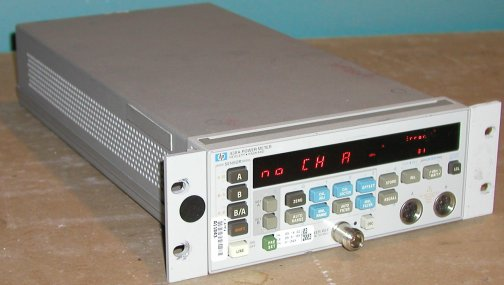The Keysight 438A dual power meter allows you to make relative measurements between two power heads (A with respect to B, or B with respect to A), without the need for scrap paper or a calculator. This feature allows you to automatically track the relationship between input and output power of a device over a range of power levels and directly read out the gain compression without plotting Pin/Pout.

Another feature of the HP438A that is quite useful is its ability to take readings relative to a reading on the same power head, that you store in memory. This is done by pushing the "REL" (relative measurement) button. Still another excellent feature is the ability to add offsets to either of the power heads. This is very useful when you have to remove losses outside your DUT from your measurements (which is almost always).

Power heads are the sensors that convert RF and microwave signals to analog voltages, which are read and reported by the power meter. There are three types of sensors in use in power heads. The first two, thermistor and thermocouple sensors, convert the "heat" of the incident signal to a voltage proportional to power. Thermistor power heads are regarded as "old-fashioned", if you see any of these in your lab they are hooked to an old analog meter such as the HP/Agilent 432A. The third type is a diode detector, which rectifies the signal into a DC voltage. Diode detectors provide a lower power range than thermistor power heads (and a lower maximum safe power), so if you are using a power head that measures down to -75 dBm, chances are you are using a diode detector power head. Another hint that you are using a diode detector is that Keysight uses the suffix "D" for diode, as in "8487D". The exception to this rule is the now-obsolete 8484A diode detector power head.

For power meter measurements, you will need to choose one or more power heads. Keysight 8480 series power heads that are commonly available are shown in the following table (some of these may be obsolete by now). It is important to stay within the "best" power response range of a power head ("throw away the bottom ten dB and top five dB of its range) . For example, if the power head is specified to for -75 to -20 dBm, don't try to use it below -65 dBm or you will experience slow settling times and flaky results.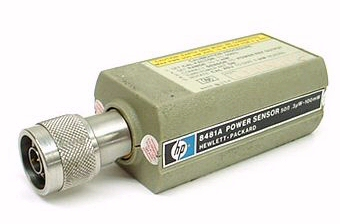Table 1. Examples of Keysight power head capabilities, 8480 series

HP model number Frequency range Specified power range (dBm) Best power range (dBm) Maximum input power (dBm) RF connector
8481A 10 MHz
to 18 GHz
-35 to +20 -25 to +15 25 N
8481H 10 MHz
to 18 GHz
-15 to +35 -5 to +25 35 N
8482A 100 KHz
to 4 GHz
-35 to +20 -25 to +15 25 N
8484A 10 MHz
to 18 GHz
-75 to -20 -65 to -25 20 N
8485A 50 MHz
to 26.5 GHz
-30 to +20 -20 to +15 25 3.5 mm
8487D 50 MHz to
50 GHz
-75 to -20 -65 to -25 20 2.4 mm
R8486A 26.5 to
40 GHz
-30 to +20 -20 to +15 25 WR-28

#### Power handling of power heads

Never exceed the maximum power rating of the power head(s) you are using. The cost to replace a power head that has been blown up by exceeding its maximum power is \$1K to \$3K, depending on which one you destroy. You can always "increase" the power range of a power head by adding an attenuator to its input, which you can remove mathematically. Be sure that the attenuator you choose can handle the power as well. Note that a "poor-man's power attenuator" can always be constructed from a coupler and a high-power load. For example, a 10 dB coupler terminated with a 10 watt load makes a 10 dB/10 watt attenuator measured through the coupled port.

### Calibration factor

Power heads convert RF power to an analog voltage, usually according to a square-law response, The calibration factor" (cal factor, or CF) of a power head is a measure of the difference between what it would ideally measure versus what it actually measures, independent of power level , versus frequency. Cal factor is typically a percentage less that 100% (like 97.5%, but in some situations cal factor can be above 100%. Your power head will have the cal factor versus frequency attached to it. Hopefully the metrology guys don't put their sticker on top of this important data!

An experience lab rat always configures a power head so that the cal factor table is readable when the measurement setup is complete. Pay attention to details like this!

Read how calibration factors are measured, here.

### Calibrating the power meter

Before you start calibrating the HP 438A power meter, hit the preset button to put the meter into a known state. This eliminates all offsets and filtering and other weird stuff from leftover from the last user that might mess with your measurement and ruin your day. According to the HP manual, the meter needs one hour to warm up to be at full accuracy. Have another donut.

Calibrating a power meter is a two step process. First, the meter should be zeroed. When you zero a meter, be sure that it has no incident RF power by turning off all sources on the bench. Both power heads should be zeroed approximately every two hours to prevent drifting. Once in a while the meter will politely ask you to "PLEASE ZERO", this is normal. Again, just be sure that there are no stray signals at the power head when you zero it.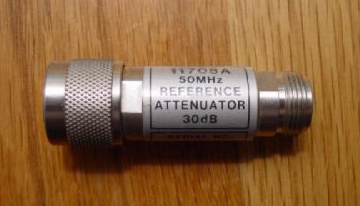Finally, you need to enter the calibration factor at the frequency of operation at which you will be testing. This information is printed on the side of the power head and is a number usually from 90% to 100%. If you use the wrong cal factor by a few percent, the error is usually small. For example, if you leave the cal factor on 100% after cal adjust but it should be set to 98% for your measurements, your data will read 0.09 dB too low (equal to 10xlog[.98]). If you want to be super accurate, you can interpolate the cal factor when you are measuring frequencies that fall between the calibration points, which are typically every gigahertz.

One thing to consider when you are taking power measurements over a wide frequency band... instead of inputting the cal factor(s) each time you change frequencies, you can leave the cal factor set at 100%, and make corrections to the data later in a spreadsheet. You should be using a spreadsheet to keep track of input and output network losses and other stuff, not to mention plotting the data, so it is no big deal to enter the cal factors here as well. Heck, you can even use it to do the pesky linear interpolation between calibrated frequency points in the spreadsheet! The way to correct data that was taken using 100% CF is to divide the measured data (in watts, or milliwatts, or microwatts, but not in dBm) by the proper cal factor at each frequency point.

### Power meter measurement errors

It is easy to take power data with a power meter. It is not so easy to take accurate, repeatable data, unless you understand all of the problems that can occur, so listen up!

#### Errors due to standing waves

A significant source of error with power meter measurements is the standing wave ratio of the power head beating against the DUT. The VSWR of the power head is usually low (less than 1.18:1 to 12.4 GHz for the 8481A power head for example). The bigger problem is usually your DUT. Suppose you are testing an amplifier with 3.0:1 VSWR (r=0.5) with a power head that has 1.18:1 (r=0.082). Because we don't have a handy Greek alphabet on this web site, just pretend that the preceding "r" was a "rho"... The high and low errors can calculated as: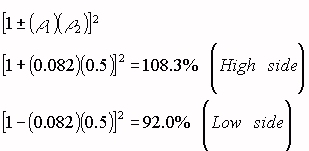An eight percent error in power is a sizable 0.36 dB error. You can avoid SWR errors if you use attenuators on both sides of your DUT to pad down its VSWRs. Of course, you will have to mathematically remove these losses from your final data.

#### Errors due to power head non-linearity

As mentioned before and described in Table 1, it is important to operate power heads within their best power range to reduce linearity errors. You can determine the linearity error of your setup by making a "through" connection in place of the DUT, displaying A/B power ratio set relative to the lowest input power of your experiment. Then sweep the input power up to the maximum power while observing the A/B reading. If the setup is done correctly you should see no more than +/- 0.1 dB errors across a power range of 20 dB or more.

A note about the above linearity test... for devices under test with substantial gain or loss, you won't be making a apples/oranges comparison of the linearity of the test setup with and without the DUT unless you put some further thought into this. If you are characterizing an amplifier with 20 dB gain, you could test the amp with a 20 dB attenuator on its output. Then the net gain, with and without the DUT will be approximately the same (zero dB), so that you can use the same power head over the same range of power. Or you could swap power heads between the calibration and measurements steps. Don't lose sleep over the "through" linearity verification, just be sure to keep the power heads within their "happy range".

Coming soon!

## Calculating a Calibration Factor

From TEGAM Application Note 218

New for September 2010! Below is a description of how calibration factors are accurately derived, thanks to TEGAM.

When using an RF Power Meter with an RF Power Sensor to make an RF Power Measurement the user must know that the measurement is accurate and there is traceability to a known standard. All diode, thermo-electric, and thermistor power sensors have calibration factors associated to particular frequencies that are used to insure an accurate power measurement. Technicians and engineers use these calibration factors when making measurements; but where do these calibration factors really come from?

Calibration factor of a terminating power sensor, if it is a DC-substitution sensor, relates the change in DC substituted power to the total RF power incident on the sensor. For this purpose, incident means all of the RF propagating toward the sensor reference plane, including power that is subsequently reflected. On the signal flow diagram, the incident power is Pi=|al|2.

Calibration factor of a feed-through power calibration setup, again if the monitor is a DC-substitution sensor, relates the change in DC substituted power in the monitor to the power delivered out of the DUT port into a load of exactly the nominal characteristic impedance of the system, or Z0. If we think of the feed-through DUT port as the output of a leveled generator, then the output into a perfect load is PgZ0.

So for a terminating sensor (using the "M" subscript following Weinschel part numbering):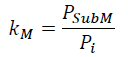And for a feed-through stand, (using "F" to indicate "feedthrough"):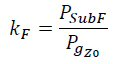Where:
kM = Calibration factor of the Terminating Mount
kF = Calibration factor of the Feed-through Mount
PSubM = Power measured terminating mount
PSubF = Power measured Feed-through mount

In all calibrations, calibration factors are transferred from a terminating reference, to a feed-through stand, and then into the DUT. In some procedures, this transfer occurs all in one session, and in others time is saved by transferring into the feed-through once and then using that feed-through calibration factor to calibrate DUT for some time.

In a perfect world, the terminating sensor would present a perfect load, and then by reorganizing the above two definitions with incident and output power equal, we would have,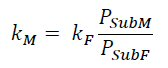Because power sensors are always imperfect loads, additional analysis and sometimes correction is required.

Figure 1 shows a signal flow diagram of two one-port devices connected together. The left-hand port is the "output", or "generator" port and is designated on the diagram using the subscript, "g". The right-hand port is the "input", or "load" port and is designated using the subscript "l". It's clear from the diagram that port reflections denominated by the Gamma vectors generally cause the power that the generator port would output into an ideal load, PgZ0, to not be equal to the power Pi, incident on the load. We really have to take into account the combination of reflections, or "port match" to come up with a general understanding of calibration transfer.

Start with the signal flow diagram. In this case, a Generator "g" is represented by an ideal source "s", and its port reflection, and the sensor is termed the load, "l".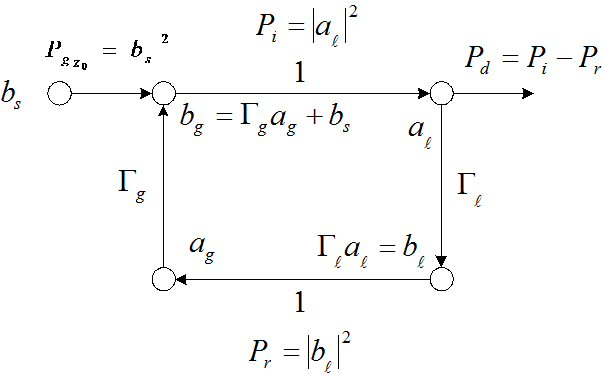Figure 1 - Signal Flow Diagram

From the diagram,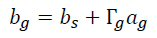But also,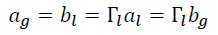So substituting the second equation into the first: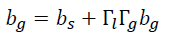And then re-arrange to collect bg: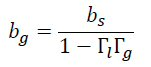Now rewrite as power, substituting in PgZ0=|bs|2, and Pi=|bg|2: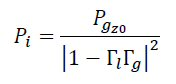Armed with this last result, and calling the Feed-through stand (F subscript) the "generator" (g subscript) and terminating sensor (M subscript) the "load" (l subscript), and substituting in the definitions for cal factor from earlier, we get the more general equation for transferring between a feed-through and a terminating sensor: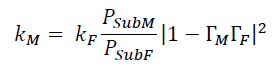Where:
km = Calibration factor of the Terminating Mount
kF = Calibration factor of the Feed-through Mount
PSubM = Power measured terminating mount
PSubF = Power measured Feed-through mount
Gamma Correction full vector data Terminating Mount
F = Gamma Correction full vector data Feed-through Mount

Now in this general equation, the Gamma terms are the reflection scattering parameter of the respective port noted in the subscript. Gamma is a complex vector with scalar values denoting the real and imaginary magnitudes: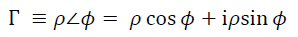In the general transfer equation, the term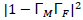is the scalar "gamma correction" or "port match" term. Inside the absolute value brackets, however, is a vector subtraction. Expanding out to make the angles explicit, this becomes: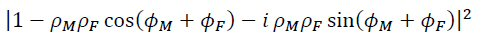Where the i represents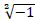, or the "imaginary" component.

The absolute value, or length of a vector, is given by the Pythagorean formula, which is the square root of the square of the magnitudes of the real and imaginary components. It's convenient that we are looking for the square of the magnitude, so we don't have to worry about the square root part. Our correction term becomes the scalar,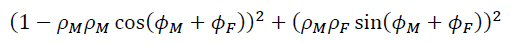When the squares are evaluated, this expands to: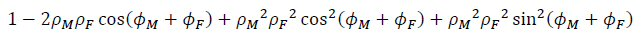Noting that if we collect the two terms beginning with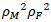, we get,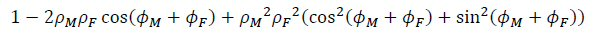The term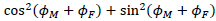, is always identically equal to 1, so the final simplified equation becomes,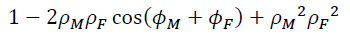Or combining this result with the general transfer equation,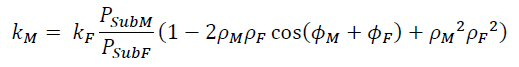If we look at the scalar result of the mismatch term, the "1" part is what would happen if at least one of the ports was "perfect", or had no reflection. In that case, one of the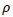is zero. The right-most element has magnitude of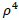, which is typically so much smaller than the middle term that it can be ignored for most connections.

The middle part,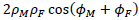, contains the bulk of the impact of port mismatch. Since it is multiplied by k, the sensitivity to this change is equal to k, or about 1. In an UNCORRECTED transfer, this part represents the probable error of the transfer. Since we can't know the angles in an uncorrected transfer, we let cos() take its limits of +/- 1, and say that the uncertainty of the uncorrected transfer is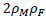. That is a little pessimistic, because that is worst-case rather than probable, but it's what the industry usually does. Unless the rhos were measured on a scalar analyzer, we have to use manufacturer's worst-case values.

Some common power sensor calibration practices do not always use gamma correction. An argument could probably be made that this was a reasonable practice at lower frequencies. We typically see this at 18 GHz and lower. A value of 0 would be inserted for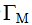making that portion of the formula "1".

This would make the formula look like: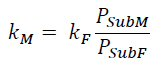This revision of the original formula assumes many things that are not necessarily true. Things that may not necessarily show up at lower frequencies but will certainly show up at higher frequencies where connectors change from the very rugged N-type connector to the more sensitive 3.5 mm and 2.4 mm connectors.

By looking at Figure 2 we can get a practical visualization of the relationship between calibration factor, gamma and effective efficiency.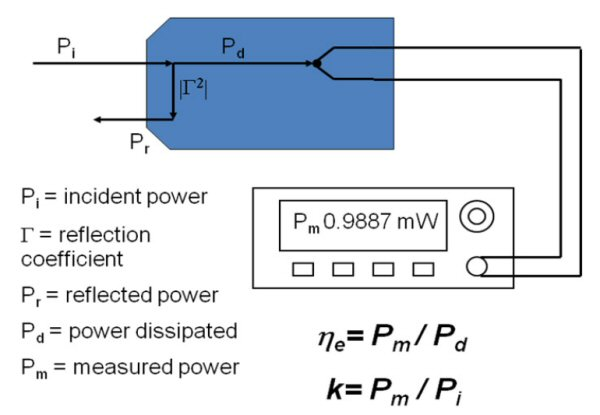Figure 2 - Relationship between Calibration Factor, Gamma, and Effective Efficiency

## The "Best Setup" for measuring power transfer characteristics

Figure 1 below shows a highly useful Pin/Pout measurement setup, based on many years of experience slaving away out in the lab. This test bench could be automated using LabView if you are so inclined, but it works quite well for taking data manually. The setup exploits the A/B relative power measurement capability of the dual power meter to quickly find compression points of your device under test (DUT) without even plotting any data. The setup can be used to measure P1dB of two-port networks (amplifier, limiter, multiplier) and as well as three-port networks (frequency translators such as mixers).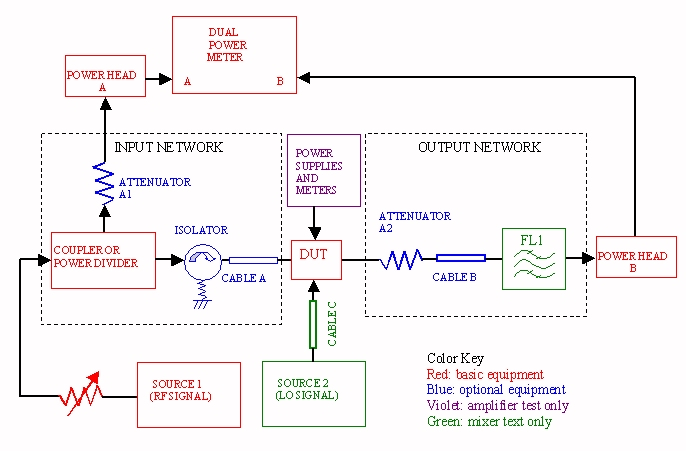Figure 1. Power test bench in operation

Referring to the figure, you'll need one RF signal source for amplifier measurements, and two for mixer measurements. Beyond the need for a second source, there are other key differences between measuring an amplifier and measuring a mixer. For example, you will have to keep track of the losses of the input and output networks as well as the power head cal factors at the different input and output frequencies (RF and LO) for mixer measurements.

Be sure that the sources you use are able to handle the RF and LO frequencies that your DUT requires. You should pick synthesized sweep oscillators over old-fashioned sweepers, so that frequency errors are minimized. Although many sources provide a built-in variable attenuator function that allows you to control the RF power level, you should consider using an external, infinitely-variable attenuator to control the signal level as shown, since this way you can quickly adjust the signal to within a few hundredths of a dB. We like to use waveguide rotary-vane attenuators for this purpose.

Signal source 1 must provide the power level needed to put the DUT well into compression, and source 2 needs to provide the proper local oscillator power level for mixer tests. For high power levels you can add power amplifiers to either source, but you may have to consider the effects of power amplifier broadband noise on the measurement, particularly if you use a traveling-wave tube (TWT). Perhaps more importantly, you will have to consider the effect of high-power signals on each and every component in the setup so that you don't roast anything. Remember, if you do barbeque a component, consider sending us a photo of the remains for the Microwave Mortuary!

Within the input network,the coupler following source 1 samples the input signal to the DUT. Attenuator A1 may be used to adjust the input power to power head A to put it into its "best" range (10 dB to 30 dB less than the maximum power). It also serves to reduce SWR errors on the input side of the DUT. The isolator that follows the input coupler helps reduce SWR errors if your DUT has a poor input match. It also prevents directivity errors, by keeping reflected power from the DUT from corrupting the power meter reading at power head A. If you use a high-directivity coupler in the input network this should take care of the problem without the need of an isolator. Be sure the coupler and isolator operate over the required bandwidth. To determine the measurement uncertainty of the input network due to directivity, you can perform the following check before you measure your DUT. Insert a matched 50 ohm load where the DUT would go and apply CW signal from source 1. Observe the power level of power head A. Now remove the matched load and attach a short circuit (if a short is not available an open circuit will be nearly as good). The maximum directivity error of the input network will be the difference in the two power meter readings. You should strive for less than 0.1 dB error here.

Cables A and B may not be necessary, they are used for convenience as well as mechanical strain relief. If you didn't use any cables at all, you could hang the coupler, DUT and both power heads of the sweeper's RF output RF connector, which might amount to 50 inch-pounds of lateral torque on the coax connection to the source. We don't have to tell you why that would be bad, do we?

In the output network, attenuator A2 can be used to adjust the power into power head B to keep it within the "sweet spot" during DUT measurements. For example, if your DUT is known to provide 30 dBm saturated output power, you might want a 20 dB attenuator on the output. Filter FL1 is extremely important for mixer measurements, it is there to reject RF and LO leakage that would corrupt the power head B reading. You need to find a filter that will pass the IF frequency and reject the RF and LO frequencies (by 30, 40, or 50 dB or more!) Don't have such a filter laying around the lab? Build one!

### Configuring the power test bench for your DUT

Before you measure the power transfer characteristics (input versus output power) of a nonlinear microwave device, you should have an idea what to expect. Check out our page on nonlinear devices.

The next two paragraphs need some further discussion, check back soon!

Let's start with the output network. What is the maximum output power of your DUT? You need to arrange the output network so that this power is about 10 dB below the specified range of your power head B. Suppose you are testing a two watt amplifier, and you have a 8481A power head (20 dBm is its highest specified power). You should choose a 2 watt, 20 dB attenuator for the output network. Then the highest power your power head will see is 13 dBm.

The amplifier you want to measure has 30 dB gain. At the saturated output power, you might expect it to have 27 dB gain. Therefore, you will need to drive it with at least 6 dBm power. Suppose that your sweeper that you will use for source 1 puts out 10 dBm. That means your input network, including cables, must have less than 4 dB loss. That should be no problem. Also, suppose that all you have for an input coupler is a 10 dB coupler. This means that when you drive the DUT with 6 dBm power, the coupled port on the coupler will see approximately -4 dBm. A good choice for the input power head would be a 8484A low-power unit (-20 dBm highest specified power). You should add a 20 dB pad to the coupled port (attenuator A1) to drop the power to the power head to -24 dBm maximum.

Before you continue, consider what the worst case power your power heads will see to make sure they are safe from being blown up. At the output, if you measure an extremely out of spec power amp that saturated at 4 watts instead of 2 watts, your power head will still only see 16 dBm. The 8481 is OK up to 25 dBm, so you are safe there. On the input, if the sweeper was cranked to its highest output power of 10 dBm, and your input network had zero loss, the 8484A power head would still only see -20 dBm through the 20 dB coupler. It takes +20 dBm to damage it. One final check... be sure that your DUT does not put any DC voltage on the input or output connections. If it does you should add a DC block at the offending port. Now you are good to go!

### Calibrating the power test bench

The setup must first be calibrated, meaning that you must determine the input coupling coefficient (we'll call it C1 here) as well as the output network loss coefficient (we'll call that C2). C1 is the difference between the input power at the DUT and the power displayed on power meter A. C1 is found by hooking up the setup as shown below in Figure 2, with power head B connected directly to the output of the input network (where your DUT will go). You will find C1 by displaying A/B for this reading. Take this data somewhere in the middle of the power range that you want to explore.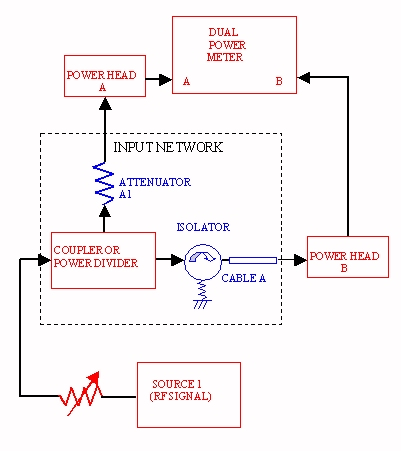Figure 2. Power test bench, input network calibration

Guess what? Only power head B needs to "speak the truth" in this measurement setup, both during the calibration and during the measurements. By this we mean that you can leave the calibration factor on power head A at 100% at all frequencies, as long as you always observe the proper cal factor on power head B during the calibration and measurement procedures at each frequency point. This is because everything that power head A measures will be relative to a measurement of power head B.

C1 should be entered as an offset to power head C1 so that the power meter will automatically display the input power to your DUT. (If you want to make this correction later in your notebook or spreadsheet, that's OK, but why not take advantage of this feature?) For example, if the coupled power is -21.3 dB less than the DUT input power, C1 is -21.3 dB, and the offset you enter for power head A is +21.3 dB. You will need to measure a coupling factor C1 at each frequency that you want to measure (and change the offset during measurements at each frequency), although over a narrow bandwidth you may not notice a big difference in C1 over frequency.

Next you need to determine the output network loss coefficient C2. For non-mixer measurements, C2 can be measured by hooking up the setup completely, but without the DUT, as shown in the figure below. The difference between the measured power at power head B and the input power measured at power head A (with its coupling factor C1 entered as an offset) will be the output loss C2.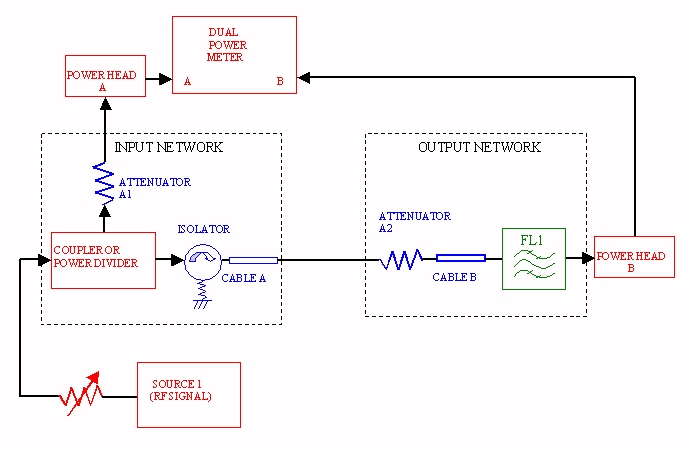Figure 3. Power test bench, output network calibration

For mixer measurements, you are better off measuring the output loss (coefficient C2) using a network analyzer. This is mainly because the input coupler will have a completely different response at the IF frequency than it did when you measured the coupling coefficient C1.

### Detailed measurement procedure example

The following measurement procedure is used for measuring a two-port device such as an amplifier. Ryan, thanks for helping us check this! Although it is not explicitly stated, it is good practice to turn off the RF power when making changes to the setup, then turn it on when you need to make a measurement.

1. Make sure that the power meter has warmed up for one hour with the power heads connected. Have another donut while you wait.
2. Hit the preset button, this eliminates all of the offsets and cal factors stored by the last guy who used the power meter.
3. Select dBm for your data, not watts (dBm is preferred by 9 out of 10 microwave engineers!)
4. Turn off all RF source power and high power amplifiers on your bench and zero the power heads. The power meter should eventually read "LOG ERROR" for both power heads.
5. Calibrate both power heads using the power meter's built-in 50 MHz reference oscillator. Attach power head A to the reference oscillator output (the type N jack on the face of the power meter), select power head A to display (hit the "A" button). Now hit "CAL ADJ", and input the cal reference setting for power head A (typically 100%). Note: for low power heads, you will need to use the Keysight 11708A precision 30 dB/50 MHz attenuator.
6. Check the cal adjustment of power head A by turning the 50 MHz oscillator on, with the cal factor set to the cal reference setting (typically 100%). You should read 0.00 dBm for all power heads except the ones that require the 30 dB reference attenuator. These power heads should read -30.00 dBm.
7. Repeat Steps 5 and 6 for power head B. Turn off the reference oscillator.
8. Enter the cal factor for power head B (output power) for the first frequency you will be measuring. Note: leave the cal factor for power head A at 100% from start to finish of this procedure.
9. Now it's time to determine input coupling coefficient C1. Attach the power heads to their proper locations in the test setup for calibrating the input network (see Figure 2). Turn on the RF power and set it at the midrange of where you plan to sweep. Display A/B on the meter. This is the input coupling coefficient at your first frequency. Write it down.
10. Determine if you have enough power range from your setup While observing power meter B (at this step it reads the input power to your DUT), vary the RF power using the sweeper's built-in attenuator and/or the external variable attenuator (if you have one). Observe that you can reach the minimum and maximum input power that your measurement requires.
11. You can now measure your worst-case directivity error. Display power meter A, then hit "REL" to reference the meter to the current power level (meter should read 0.00 dB). Then briefly remove power head B and replace it with a short circuit. If you have excellent directivity, the power meter reading should only change by perhaps 0.1 dB. Write it down. Put power head B back and continue.
12. Repeat steps 8, 9 and 10 for each frequency you will be measuring, and create a table for the input coupling coefficient C1 over the frequency range. You can skip the directivity error measurement (step 11) on the additional frequencies if you are confident that your setup has good directivity at each frequency. Don't forget to change the power head B cal factor when you change frequencies.
13. Now it's time to measure the output loss coefficient C2Note: in the case that your output network has no appreciable loss, you can skip this step. Connect up the test bench without the DUT, as shown in Figure 3. For the first frequency, enter the input network coefficient C1 as an offset to power head A, and be sure to set the proper calibration factor for power head B. Turn on the RF and set it near your midrange power level. Due to the C1 offset, power head A is now reading your DUT input power directly. Display A/B on the power meter; this is equal to your output network loss coefficient C2. Write it down.
14. Repeat step 13 for each frequency that you will be measuring, and create a table for C2 over the frequency range.
15. Now it's time to measure the power transfer characteristics of your DUT. For each frequency, enter the proper cal factor for power head B, enter the input coupling coefficient C1 as an offset to power head A, and enter the output loss coefficient C2 as an offset to power head B. Install the DUT and bias it to the target quiescent point. Turn on the RF, and set it to the lowest power setting you want to take data at. You are now good to go!
16. You now have your choice of displaying input power (power meter A), output power (power meter B) or gain (A/B). You only need to write down two of these, we like to record input power and gain. Using steps of approximately 1 or 2 dB, measure the input power, gain and drain current IDS for the amplifier. Start at 20 dB below the P1dB point on the data sheet and continue until the amplifier output is saturated (one dB change in Pin results in less than 0.1 dB change in Pout).
17. Using Excel, enter the data and plot output power, gain and power-added efficiency versus input power (see below).

### Finding compression points quickly

If all you are interested in is finding gain compression points such as the one-dB compression point (P1dB) of your DUT, you can do this quickly and directly. Following step 15 above, set the meter to display gain of the DUT (A/B). Now hit the "REL" button to reference the gain to the current reading. It should now read 0.00 dB. Now all you have to do is decrease the attenuation (increasing the input power) until the meter reads -1.00 dB, and voila, you are at the P1dB point! Display the P1dB(in) by selecting power meter A, or P1dB (output) by selecting power meter B.

### Plotting power transfer characteristics

Go to our separate page on compression point.

### Plotting amplifier efficiency

When you measure the power transfer characteristics of an amplifier, you can calculate efficiency (PAE or drain) at the same time if you record the DC input power (voltages and currents).

More coming soon!

## Measuring return loss with power meters

Coming soon!

This page is now sponsored by our friends over at TEGAM, near Cleveland Ohio. Need some expertise in power measurements? Check them out!

Author : Unknown Editor